+
Introduction to Arithmetic Sequences
Solving Linear Equations and Inequalities
0
of 0 possible points

# Introduction to Arithmetic Sequences

Author: Sophia Tutorial
##### Description:

Calculate the value of the nth term or the term number that has a specific value in an arithmetic sequence.

(more)

Sophia’s self-paced online courses are a great way to save time and money as you earn credits eligible for transfer to many different colleges and universities.*

No credit card required

37 Sophia partners guarantee credit transfer.

299 Institutions have accepted or given pre-approval for credit transfer.

* The American Council on Education's College Credit Recommendation Service (ACE Credit®) has evaluated and recommended college credit for 33 of Sophia’s online courses. Many different colleges and universities consider ACE CREDIT recommendations in determining the applicability to their course and degree programs.

Tutorial
what's covered
1. Sequences
2. Arithmetic Sequences
3. The Formula for Finding a Term in an Arithmetic Sequence
4. Writing a Formula for an Arithmetic Sequence
5. Using the Formula to find an and n

# 1. Sequences

In math, a sequence is a set of numbers in a particular order. For example, {1, 3, 5, 7, 9} is a sequence, because we have numbers in a set (denoted by the curly braces) and the numbers are in numerical order. This particular sequence is finite, because there are a limited number of terms (there are only 5 numbers). Sequences can be infinite, meaning that they are an endless number of terms. {1, 3, 5, 7, 9...} is an infinite sequence, denoted by the ellipsis (the "dot-dot-dot"). This means that the sequence continues to include all positive odd integers.

# 2. Arithmetic Sequences

The example sequence above also happens to be an example of an arithmetic sequence. Like all sequences, it is a set of numbers in numerical order. What makes it an arithmetic sequence is the constant change in value from one term to the next. In the sequence {1, 3, 5, 7, 9...}, we add 2 to each term to get the value of the term after it. This constant change in value is called the common difference between terms. Let's define a few things about arithmetic sequences:

terms to know
Arithmetic sequence
a set of numbers in numerical order, with a common difference between each term.
Term (in a sequence)
refers to the place or order of a number in a sequence, such as first, second, third, etc.
Common difference
the numerical distance between any two consecutive terms in an arithmetic sequence, a constant value.

Now that we know that {1, 3, 5, 7, 9...} is an arithmetic sequence with a common difference of 2, what are the values of the next two terms in the sequence? We just continue to add 2 to the last term we know. The value of the next two terms are 11, and 13.

# 3. The Formula for Finding a Term in an Arithmetic Sequence

It was easy enough to find the value of the next two terms in the sequence above, because we could just add two a few times. What if we wanted to find the value of the 50th term? Or the 400th term? Certainly adding two by hand hundreds of times isn't the easiest way. Instead, we can use this formula:

formula
Arithmetic Sequence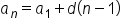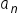The value of the nth term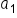The value of the 1st term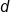Common difference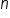Term

Next, we are going to use this formula to write formulas for specific arithmetic sequences, and then solve for the value of the nth term, as well as find n given its value.

# 4. Writing a Formula for an Arithmetic Sequence

Consider this sequence:

 {7, 11, 15, 19, 23, 27, 31, ... }

How can we write the formula to describe the value of any term in this sequence? We know that part of the formula is the value of the first term, so we know that 7 will be included in the formula. The biggest thing is to find the common difference. Remember that the common difference the the numerical distance between any two consecutive terms in an arithmetic sequence. So just pick two terms that are next to each other, and subtract one from the other. Let's choose the terms 23 and 19. 23 – 19 = 4.

hint
It is a good idea to check that the common difference is 4 throughout the sequence (in case what we are working with isn't arithmetic at all! Also keep in mind that the common difference may be negative; this is the case when the terms are decreasing in value rather than increasing.

So we have our common difference of 4, and the initial term is 7. Let's put this into our formula: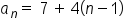# 5. Use the Formula to find an and n

Now that we have a formula to describe the arithmetic sequence above, we can use it to find the value of the nth term, as well as find the term number that has a specific value. First, let's find the value of the 18th term.

Recall that the variable n stands for the term number. So to find the value of the 18th term, we substitute 18 in for n and solve: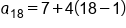Substituting 18 in for n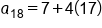Evaluate 18-1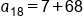Evaluate 4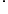17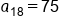Our Solution

Next, let's use the formula to find the term that has the value of 255. Here, we need to solve for n, given that a(n) = 255.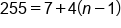Substitute 255 in for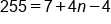Distribute 4 into (n-1)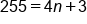Combine 7 and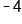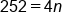Subtract 3 from both sides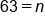Our Solution

This means that the value of the 63rd term in the arithmetic sequence is 255.

summary
In math, a sequence is a set of numbers in a particular order. We defined an arithmetic sequence as a set of numbers in numerical order with a common difference. The common difference is the numerical distance between any two consecutive terms in an arithmetic sequence. We can use the formula for finding a term in an arithmetic sequence to find larger terms in the sequence, such as the 50th or 400th term. We can use this formula when writing a formula for an arithmetic sequence. We can also use the formula find a(n) or n.
Terms to Know
Arithmetic Sequence

a set of numbers in numerical order, with a common difference between each term

Common Difference

the numerical distance between any two consecutive terms in an arithmetic sequence; a constant value

Term (in a sequence)

refers to the place or order of a number in a sequence, such as first, second, third, etc.

Formulas to Know
Arithmetic SequenceRating